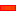﻿ Getting Started Tutorials - MechDesigner > Tutorial 15: Patterns - Indexing-Chain example > Step 15.2: Design the Motion for the Belt

# Step 15.2: Design the Motion for the Belt

### Design the Motion for the Belt.

We need to design the motions a Point on a Chain as it moves around two Pulleys.

The Point moves in an arc as it moves around a pulley.

The Point moves in a straight line as it moves between the pulleys.

Design decisions we have made for the Belt:

The belt has twelve (12) pockets

Each pocket and tool has a pitch of 100mm

Thus, the belt is exactly 12 x 100mm = 1200mm long.

Each Pulley has five teeth.

Hence:

oCircumference of each Pulley = 500mm, Radius = 500/2π = 79.577mm

oDistance between Pulleys = (1200 – 500)/2 = 350mm

 Note: All of the calculations we do in this step can be done much more easily with a: •a sketch to define the path of the Belt,•A Motion-Path FB will add a Motion-Point to the belt sketch, •a motion can be defined for the Motion-Point. at the input-connector to the Motion-Path FB.However, the information below helps us understand the how to edit the Sinusoid Segment.

####Motion Design Preparation and Calculations for the Piggyback SlidersHow to Find the Phase Parameter for the Sinusoid SegmentsComplete the Model in MechDesigner